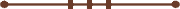####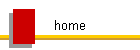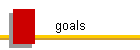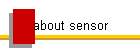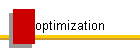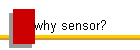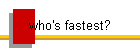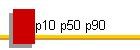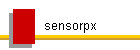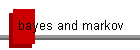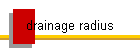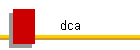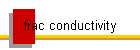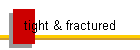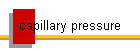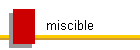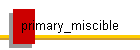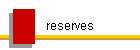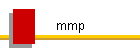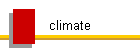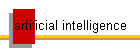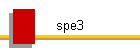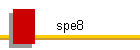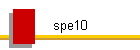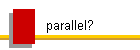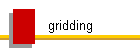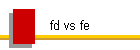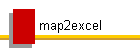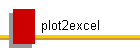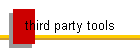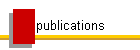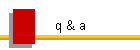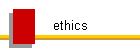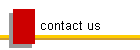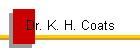### Bayes' Theorem and Markov Chain Theory

Also see:

Bayes' theorem and Markov Chain theory are increasingly used to treat the problems of uncertainty quantification and optimization in reservoir characterization and simulation (search One Petro for "Bayes Theorem" and "Markov Chain").

The Petroleum Engineering Handbook does not define Bayes' theorem.

"The formula is written as P(A|B) = P(B|A) * P(A) / P(B). P(A) and P(B) are the probabilities of A and B without regard to each other. P(B|A) is the probability that B will occur given A is true. Finally, the answer, P(A|B) is the conditional probability of A occurring given B is true."

The condition applying to the theorem is P(B) > 0.

In reservoir characterization and simulation and all applications such as reserves estimation, there is no such thing as an anterior or a posterior probability and Bayes' theorem does not apply, because P(A) and P(B) are both 0, due to the very large number of unknown variables. That number is generally equal to many times the numbers of gridblocks and wells.

For any given real input in a simulation model of a real reservoir, what is the probability that it is exactly correct? (0) I'm thinking of a real number between 0 and 1. What is the probability that anyone can guess what it is? (0) What is the probability that any given reservoir realization is correct? (0). If a given realization is found to better match history, what is it's probability of being correct? (0) If a given parameter in a given realization is adjusted to better match history, what is the probability that the right parameter to adjust is selected, and that the correct adjustment is applied to it? (0)

Markov chain theory defines the probability of a modified realization from the probability of an existing one.  Since those probabilities are generally both 0 in reservoir simulation due to very large numbers of uncertainties, the theory does not apply.

Therefore, to answer any question by reservoir characterization and modeling when there is significant uncertainty in the inputs, some number of equally (im)probable realizations must be used in probabilistic analysis.

© 2000 - 2022 Coats Engineering, Inc.